Moduli of a Riemann surface

(diff) ← Older revision | Latest revision (diff) | Newer revision → (diff)
Numerical characteristics (parameters) which are one and the same for all conformally-equivalent Riemann surfaces, and in their totality characterize the conformal equivalence class of a given Riemann surface. Here two Riemann surfacesand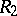are called conformally equivalent if there is a conformal mapping from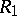onto. For example, the conformal classes of compact Riemann surfaces of topological genus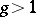are characterized byreal moduli; a Riemann surface of torus type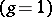is characterized by 2 moduli; an-connected plane domain, considered as a Riemann surface with boundary, is characterized bymoduli for. About the structure of the moduli space of a Riemann surface see Riemann surfaces, conformal classes of.
A necessary condition for the conformal equivalence of two plane domains is that they have the same connectivity. According to the Riemann theorem, all simply-connected domains with more than one boundary point are conformally equivalent to each other; each such domain can be conformally mapped onto one canonical domain, usually taken to be the unit disc. For-connected domains,, a precise equivalent of this Riemann mapping theorem does not exist: It is impossible to give any fixed domain whatever onto which it is possible to univalently and conformally map all domains of a given order of connectivity. This has led to a more flexible definition of a canonical-connected domain, which reflects the general geometric structure of this domain, but does not fix its moduli (see Conformal mapping).
Each doubly-connected domainof the-plane with non-degenerate boundary continua can be conformally mapped onto some circular annulus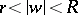,. The ratioof the radii of the boundary circles of this annulus is a conformal invariant and is called the modulus of the doubly-connected domain. Letbe an-connected domain,, with a non-degenerate boundary.can be conformally mapped onto some-connected circular domain, which is a circular annuluswith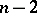discs with bounding circles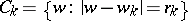,, removed; the circles,, lie in the annulusand pairwise do not have points in common. Here it can be assumed thatand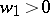. Thendepends on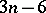real parameters: the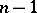numbers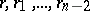and the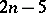real parameters defining the centres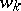of the circles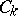,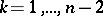. Thesereal parameters can be taken as moduli of the-connected domainin the case.
As moduli of-connected domainsit is also possible to take any otherreal parameters (if, and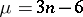if) which determine a conformal mapping ofonto some canonical-connected domain of another shape.